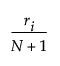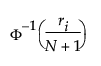Publication date: 11/10/2021

## Normal Quantile Plot

The empirical cumulative probability for each value is computed as follows:where ri is the rank of the ith observation, and N is the number of nonmissing (and nonexcluded) observations.

The normal quantile values are computed as follows:where Φ is the cumulative probability distribution function for the normal distribution.

These normal quantile values are Van Der Waerden approximations to the order statistics that are expected for the normal distribution.